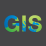# How to use query in arcpy.Append_management

941
2
06-13-2019 07:01 PMOccasional Contributor

it's weird that the arcpy.Append_management function does not have any arguments for SQL Expressions.

Does anyone know a way around this ?

Below is my code. Basically I want to take a feature layer with a sql statement and put it in a sde.

Would I have to use arcpy.MakeFeatureLayer_management() then append?

And I want to ensure that the expression only grabs 1 feature, if there are more than 1 features trying to append it will fail. Would I do this in the validation or could I do this in the script ?

``````import arcpy, os, datetime, sys

arcpy.env.overwriteOutput = True
scratchLoc = arcpy.env.scratchFolder
cs= arcpy.SpatialReference(102003)
xmlLoc = r"\\......_Scripts\KMZtoInvBdy\INV_BDY.XML"
gdbname = r"prjbdy_scratch.gdb"
fcLoc = r"\prjbdy_scratch.gdb\inv_project_boundary"
cntylyr = r"......\Default.gdb\AA_counties_SpatialJoin"

mxd = "CURRENT"
date = datetime.datetime.now()
dateprj= date.strftime("%d/%m/%Y")

fl = arcpy.GetParameterAsText(0)

Expression = arcpy.GetParameterAsText(1)
if Expression == '#' or not Expression:
Expression = "" # provide a default value if unspecified

Project_Name = arcpy.GetParameterAsText(2)

Project_Status = arcpy.GetParameterAsText(3)
if Project_Status == "Operating":
Project_Status = "OPE"
elif Project_Status == "Prospecting":
Project_Status = "PRO"
elif Project_Status == "Development":
Project_Status = "DEV"
elif Project_Status == "Interconnection Area":
Project_Status = "INT"

Project_Tech = arcpy.GetParameterAsText(4)
if Project_Tech == "Wind Farm":
Project_Tech = "WND"
elif Project_Tech == "Solar Plant":
Project_Tech = "SOL"
elif Project_Tech == "Thermal Plant":
Project_Tech = "THM"
elif Project_Tech == "Battery Storage":
Project_Tech = "BAT"
elif Project_Tech == "Co-Generation":
Project_Tech = "COG"
elif Project_Tech == "Desalinization Plant":
Project_Tech = "DES"

Primary_Expasion = arcpy.GetParameterAsText(5)
if Primary_Expasion == "Primary":
Primary_Expasion = 1
elif Primary_Expasion == "Expansion":
Primary_Expasion = 2

MapFeature = arcpy.GetParameterAsText(6)
if MapFeature == "Display":
MapFeature = 1
elif MapFeature == "Do Not Display":
MapFeature = 0

arcpy.CreateFileGDB_management(scratchLoc,gdbname)
gdbloc = os.path.join(scratchLoc,gdbname)

arcpy.ImportXMLWorkspaceDocument_management(gdbloc,xmlLoc)

arcpy.Append_management(fl,scratchLoc + "\\" + gdbname + r"\inv_project_boundary","NO_TEST",Expression)

#be able to import any type of feature eg.shapefile,fc,geojson
#different conversions

#Calculates Area

arcpy.MakeFeatureLayer_management(scratchLoc + fcLoc, "prjbdy_Layer")
arcpy.MakeFeatureLayer_management(cntylyr, "cntlyr_Layer")
arcpy.SelectLayerByLocation_management("cntlyr_Layer","INTERSECT","prjbdy_Layer")
scur = arcpy.SearchCursor("cntlyr_Layer")
try:
for row in scur:
cnty_name = str(row.getValue("NAME"))
statename = str(row.getValue("STATE_ABBR"))
scur2 = arcpy.SearchCursor("prjbdy_Layer")
for row in scur2:
Acres = row.getValue("AREA_GEO")
del scur
del scur2
except:
#arcpy.Append_management(scratchLoc + fcLoc, scratchLoc + "\\" + gdbname + r"\inv_project_boundary","NO_TEST")

ucur = arcpy.da.UpdateCursor(scratchLoc + "\\" + gdbname + r"\inv_project_boundary",["PROJECT","PROJ_STATUS","TECH","MAP","PRIMARY_","DATE_REVISED","COUNTY","STATE","ACRES"])
try:
for row in ucur:
row= Project_Name
row = Project_Status
row = Project_Tech
row = MapFeature
row = Primary_Expasion
row = dateprj
row = cnty_name
row = statename
row = Acres
ucur.updateRow(row)
del ucur
except:

arcpy.AddMessage("County, State: " + cnty_name + ", " + statename)
'''
arcpy.Append_management(scratchLoc +r"\kml_scratch.gdb" + r"\inv_project_boundary", r"..........._project_boundary")
Tags (3)
1 Solution

Accepted SolutionsbyEsri Esteemed Contributor

Would I have to use arcpy.MakeFeatureLayer_management() then append?

Yes, this correct.  You will then pass the Feature Layer as the input for the Append.  Before doing so you can execute Get Count function and only run the Append if the count is equal to one.  Ex:

``````arcpy.MakeFeatureLayer_management(fc, "fLayer", "OBJECTID = 1")
result = arcpy.GetCount_management("fLayer")
count = int(result.getOutput(0))

if count == 1:
arcpy.Append_management("fLayer", target",....)‍‍‍‍‍‍``````
2 RepliesbyEsri Esteemed Contributor

Would I have to use arcpy.MakeFeatureLayer_management() then append?

Yes, this correct.  You will then pass the Feature Layer as the input for the Append.  Before doing so you can execute Get Count function and only run the Append if the count is equal to one.  Ex:

``````arcpy.MakeFeatureLayer_management(fc, "fLayer", "OBJECTID = 1")
result = arcpy.GetCount_management("fLayer")
count = int(result.getOutput(0))

if count == 1:
arcpy.Append_management("fLayer", target",....)‍‍‍‍‍‍``````Occasional Contributor

Thanks Jake.

Thats exactly what I did. It worked!